# Deriving Faraday’s law of induction

Faraday’s law of induction is the final law that makes up the bulk of all 4 Maxwell’s equations of classical electromagnetism. In concise terms: this law tells us how magnetic fields interact with an electric circuit to produce an electromotive force (EMF). A very important consequence of this law is that it explains the operating principle behind many electrical devices and components used everywhere in the modern age, including inductors, transformers, electric motors and generators. In this article, we will derive Faraday’s law of induction using mathematical techniques from vector calculus.

# Deriving the equation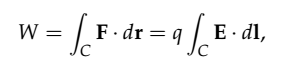Equation 1

where F = qE is the electrostatic force on a charge q and dl is an element of length along the path C. Now, we define the work done per unit charge as the potential, or voltage

We assume now that the electric field E is conservative, meaning that

this allows us to write the electric field as the gradient of some scalar field, namely

The reason this is useful is because we can now write, according to the fundamental theorem of line integrals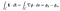Equation 2: application of the fundamental theorem of line integrals

Additionally, we take advantage of the fact that the voltage is simply the difference in potential between two points a and b

so under these assumptions, this implies that

If we now define the magnetic flux through a surface as

then, for a closed electric circuit, we define the electromotive force (EMF) as the rate of change of the magnetic flux (with respect to time)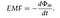Equation 3: EMF and magnetic flux

Since the EMF is also given by the voltage in a closed circuit, we can write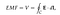Equation 4: EMF and voltage

thus equating Eq(3) and Eq(4) yields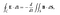Equation 5

we can eliminate these integrals by applying Stokes’ theorem on the left-hand side, such that

and finally, by dropping the surface integral from both sides we arrive at Faraday’s law of induction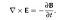Equation 6: Faraday’s law of induction in differential form

Oscar is a physicist, educator and STEM enthusiast. He is currently finishing a PhD in Theoretical Physics with a focus on photonics and stochastic dynamics.

## More from Oscar Nieves

Oscar is a physicist, educator and STEM enthusiast. He is currently finishing a PhD in Theoretical Physics with a focus on photonics and stochastic dynamics.